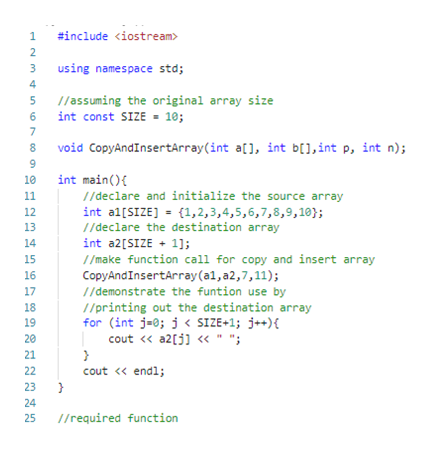# In C++Create a function called CopyAndInsertArray.  It should take in two arrays (the first array should be of size n and the second array should be of size n+1).  The function should also take in a position and a number. It should copy the first array into the second array and place the new number at the proper position.  In other words, you are creating a function that inserts a value into an array.

Question
36 views

In C++

Create a function called CopyAndInsertArray.  It should take in two arrays (the first array should be of size n and the second array should be of size n+1).  The function should also take in a position and a number. It should copy the first array into the second array and place the new number at the proper position.  In other words, you are creating a function that inserts a value into an array.

check_circle

Step 1

Program design

The overall program design is as follows:

• The function CopyAndInsertArray would accept 4 parameters – source array, destination array, insert position and element to be inserted
• the function would use a insert (or shift) status flag to indicate if we are past the insert position
• using the insert / shift status flag we undertake the copy operation
• elements before the insert position are copied into the same index of the destination array, elements after the insert position are copied into a shifted index position in the destination array
• the main function tests the use of the function in the given C++ program

The program logic is explained in detail in the embedded program comments.

Step 2

Program

#include <iostream>

using namespace std;

//assuming the original array size

int const SIZE = 10;

void CopyAndInsertArray(int a[], int b[],int p, int n);

int main(){

//declare and initialize the source array

int a1[SIZE] = {1,2,3,4,5,6,7,8,9,10};

//declare the destination array

int a2[SIZE + 1];

//make function call for copy and insert array

CopyAndInsertArray(a1,a2,7,11);

//demonstrate the funtion use by

//printing out the destination array

for (int j=0; j < SIZE+1; j++){

cout << a2[j] << " ";

}

cout << endl;

}

//required function

void CopyAndInsertArray(int a[], int b[],int p, int n){

//declare array index for manipulation

int i;

//declare flag for indicating shift status

//will be true from insert position onwards

//initialzied to false value

bool shifted = false;

//iterate over the source array elements

for (i = 0; i < SIZE; i++){

//do the following if the cuurent array element is

//before or at the insert position

if(!shifted){

//if the current element is at

//the insert position

if (i == p - 1){

//assign the current position

//of the destination array with the

//element to be inserted

b[i] = n;

//turn shift status flag to true

shifted = true;

//assign the element of the current position

//in the source array to the next position

//of the destination array

b[i+1] = a[i];

}

//if the current position is not at the

//insert position but any position before it

else

b[i] = a[i];

}

//if the current position is beyond the insert position

//i.e. shift status is true

//then assign the current (i)-th element of the source array

//to the position of the destination incremented by 1 position

//i.e. to (i+1)-th position

else

b[i+1] = a[i];

}

}

Step 3

Program im...help_outlineImage Transcriptionclose#include using namespace std 4 /assuming the original array size int const SIZE 10; 6 7 8 CopyAndInsertArray(int a[], int b[],int p, int n); int main() //declare and initialize the source array 10 11 int al[SIZE] {1,2,3,4,5,6,7,8, 9, 10}; 1/declare the destination array int a2[SIZE 1]; /make function call for copy and insert array 12 13 14 15 CopyAndInsertArray (a1,a2,7,11); //demonstrate the funtion use by //printing out the destination array (int j-0; < SIZE+1; j){ cout << a2[l " "; 16 17 18 19 20 21 22 cout << endl; 23 } 24 /required function 25 fullscreen

### Want to see the full answer?

See Solution

#### Want to see this answer and more?

Solutions are written by subject experts who are available 24/7. Questions are typically answered within 1 hour.*

See Solution
*Response times may vary by subject and question.
Tagged in

### Programing Language